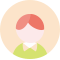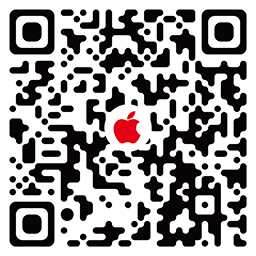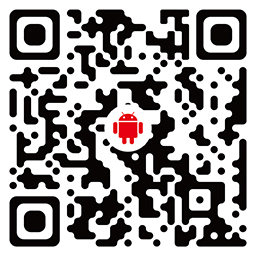Hi, 欢迎访问门门•IOS
•Android

• 云腾
• 徕图
• 曼富图
• 索尼
• 佳能
• 百诺
• 思锐
• 金蝌灵跃
• Lzqly
• 富图宝
• 多宝莱
• 栩傲宏
• 捷信
• Miliboo
• Ulanzi
• 神牛

• 三脚架
• 脚架+云台套装
• 云台
• 脚架配件
• 三脚架云台套装
• 自拍杆
• 警示牌
• 伸缩
• 落地
• 轻巧便携
• 水平仪
• 快装板
• 摄像视频类
• 桌面
• 直播设备
• 影棚图片类
• 独脚架
• 影棚拍摄道具
• 支架
• 手电
• 单镜头套装
• 机身
• 高端专业
• 夜钓灯

• 通用
• 手机
• 相机
• 滚筒
• 单反
• 单反相机
• 尼康佳能索尼
• 单反相机尼康佳能索尼
• 微单
• 所有机型
• 三脚架
• 手机/平板
• 通用型
• 任意机型
• 单反微单
• 所有
• 数码相机
• 苹果
• 单反/摄像机
• 单反摄像机
• 手机单反相机
• 摄像机
• 安卓苹果通用
• 相机通用

• 直播
• 摄影
• 桌面
• 客厅
• 摄像
• 骑行
• 家用
• 抖音/直播/户外
• 黑坑
• 旅行
• 自拍
• 书房
• 户外
• 手机k歌
• 拍摄
• 风光摄影
• 床头
• 抖音
• 主持/舞台演出
• 居家
• 通用
• 人物摄影
• 家庭ktv
• 庭院

• 2kg-5kg
• 2kg以下
• 20kg及以上
• 6kg-10kg
• 6-10kg
• 11kg-20kg
• 2-5kg
• 11-20kg
• 21kg以上
• 10kg
• 1.5kg
• 3kg
• 1kg
• 200克
• 8kg
• 500g
• 5kg
• 0.5kg
• 11-15kg
• 1kgkg
• 4kg
• 最大负载约 1kg

• 黑色
• 白色
• 黄色
• 红色
• 银色
• 灰色
• 碳纤维本色
• 绿色
• 象牙白
• 单机位+收纳袋
• 粉色
• 1
• 原色
• 蓝色
• 三脚架
• +
• 墨黑色
• rp+套机
• 三脚架自拍杆
• 雅黑色
• 云台
• 摄像机
• 天空蓝
• 酒红色

• 球型云台
• 液压云台
• 普通云台
• 三维云台
• 三轴云台
• 摄像机云台
• 全景云台
• 无云台
• 球形云台
• 悬臂云台

• 铝合金
• 碳纤维
• 金属
• 塑料
• 木质
• 不锈钢
• 合金
• 镁合金
• 搪瓷
• 纸质
• PVC
• ABS
• PU
• 实木
• 铁艺
• 铝镁合金
• 玻璃
• 玉石
• 陶瓷
• 冷轧钢
• 木质三脚架花篮一对
• 碳钢
• 藏银

-

• 1
• 2
• 3
• 4
• 5
• 6
• 7
• 8
• 9
• 10